Overview: QP Solver

The OPTMODEL procedure provides a framework for specifying and solving quadratic programs.

Mathematically, a quadratic programming (QP) problem can be stated as follows: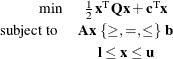where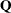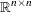is the quadratic (also known as Hessian) matrix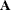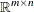is the constraints matrix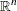is the vector of decision variablesis the vector of linear objective function coefficients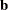is the vector of constraints right-hand sides (RHS)is the vector of lower bounds on the decision variablesis the vector of upper bounds on the decision variables

The quadratic matrixis assumed to be symmetric; that is,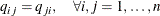Indeed, it is easy to show that even if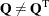, then the simple modification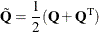produces an equivalent formulation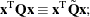hence symmetry is assumed. When you specify a quadratic matrix, it suffices to list only lower triangular coefficients.

In addition to being symmetric,is also required to be positive semidefinite for minimization type of models: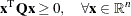is required to be negative semidefinite for maximization type of models. Convexity can come as a result of a matrix-matrix multiplication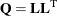or as a consequence of physical laws, and so on. See Figure 9.1 for examples of convex, concave, and nonconvex objective functions.

Figure 9.1: Examples of Convex, Concave, and Nonconvex Objective Functions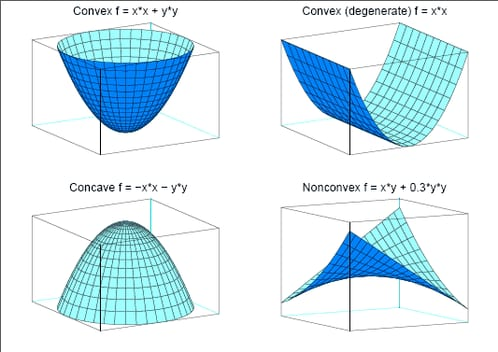The order of constraints is insignificant. Some or all components ofor(lower and upper bounds, respectively) can be omitted.